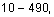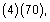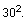# Online Calculator Tutorial

An online calculator is part of the testing software for GACE® assessments that require the use of a graphing or scientific calculator. Free tutorials are available so you can practice using these calculators prior to taking an assessment.

The following tests include the use of an online calculator:

• Mathematics Test I (022) — graphing calculator
• Mathematics Test II (023) — graphing calculator
• Mathematics Combined Test I & II (522) — graphing calculator
• Middle Grades Mathematics (013) — graphing calculator
• Special Education Mathematics and Science (088) — scientific calculator

Click on the link below to navigate to an external site where you can download the graphing and scientific calculator tutorials.

## Four-function Online Calculator

The GACE Program Admission – Mathematics test and the Program Admission – Combined test both have a four-function calculator built into the testing software. Here are some helpful tips for using the four-function online calculator:

• Most of the questions requiring the use of the four-function online calculator don't require difficult computations, so don't use the calculator just because it's available.
• Use it for calculations that you know are tedious, such as long division; square roots; and addition, subtraction, or multiplication of numbers that have several digits.
• Avoid using it for simple computations that are quicker to do mentally, such asand• Avoid using it to introduce decimals if you are asked to give an answer as a fraction.
• Some questions can be answered more quickly by reasoning and estimating than by using the calculator.
• If you use the calculator, estimate the answer beforehand so you can determine whether the calculator's answer is "in the ballpark." This may help you avoid key-entry errors.Need Help?

Chat with one of our Customer Service Representatives.

Hours:
M–F, 8 a.m.–5 p.m. ET